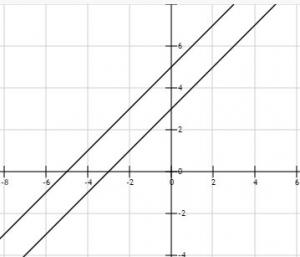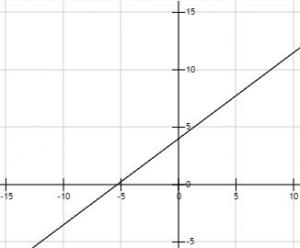# Let's Get Started

We're going to learn to use a variety of methods to solve a system of equations.

TEKS Standards and Student Expectations

A(3) Linear functions, equations, and inequalities. The student applies the mathematical process standards when using graphs of linear functions, key features, and related transformations to represent in multiple ways and solve, with and without technology, equations, inequalities, and systems of equations. The student is expected to:

A(3)(F) graph systems of two linear equations in two variables on the coordinate plane and determine the solutions if they exist

A(3)(G) estimate graphically the solutions to systems of two linear equations with two variables in real-world problems

A(5) Linear functions, equations, and inequalities. The student applies the mathematical process standards to solve, with and without technology, linear equations and evaluate the reasonableness of their solutions. The student is expected to:

A(5)(C) solve systems of two linear equations with two variables for mathematical and real-world problems

Resource Objective(s)

Given verbal and/or algebraic descriptions of situations involving systems of two variable linear equations, the student will solve the system of equations.

Essential Questions

How can a system of equations be solved by graphing?

How can a system of equations be solved by substitution?

How can a system of equations be solved by elimination?

Vocabulary

# Methods for Solving Systems of Equations Algebraically

There are essentially three different methods to solve systems of equations algebraically. They are listed and described briefly below.

The Graphing Method: When there is one variable solved in both equations, it is easy to use a graphing calculator. In this case, the calculator can be used to graph both equations. The intersection of the two lines will represent the solution to the system of equations.

m = -3n
m = 10 – 4n

The Substitution Method: There are two different types of systems of equations where substitution is the easiest method.

Type 1: One variable is by itself or isolated in one of the equations. The system is solved by substituting the equation with the isolated term into the other equation:

x + 2y = 7
y = x – 5

Type 2: One variable can be easily isolated. The systems are solved by solving for one variable in one of the equations, then substituting that equation into the second equation. Solve for a in the second equation, then substitute the second equation into the first.

2a + 3b = 2
a – 2b = 8

The Elimination Method: Both equations are in standard form: Ax + By = C. The system of equations are solved by eliminating a variable and solving for the remaining variable. Add the two equations together to eliminate the y, then solve for x.

8x + 11y = 37
2x – 11y = -7

Any of the other methods can be used to check your answer, or you can plug in the x and y values to insure that both equations give you true statements.

# Solving Systems of Equations Algebraically by Graphing

One way to solve systems of equations algebraically is by graphing. A graphing calculator is needed. If you need an online graphing calculator click here.

If the two linear equations are both equal to the same variable, you do not need to manipulate the equations. You can graph the equations on your graphing calculator as they are written, and then find the solution.

Remember, this method only works if the two equations are equal to the same variable.

Example

Solve the following systems of equations.

y = 2x – 2
y = 4x4

Put both equations into the "Y=" equation editor of the calculator: Y1 = 2x – 2 and Y2 = 4x – 4.

Check for where the graphs intersect, and answer the following questions.

# Solving Systems of Equations Algebraically by Substitution

There are two types of situations involving substitution.

Type 1

Is one of the equations written with one of the variables on one side of the equation? If so, you can substitute that variable's value into the other equation and solve it.

Example

Solve the following system of equations using substitution.

b = 3
4a + b = 7

Type 2

If one of the equations is not written with one variable alone on one side of the equation, but it is easy to transform into an equation with one variable on one side, substitution can still be used.

Example

Rewrite the equation, and then substitute it into the other equation to solve the system of equations.

2a + 3b = 2
a – 2b = 8

# Solving Systems of Equations Algebraically by Elimination

When graphing or substitution cannot be easily done, elimination is used to solve a system of equations.

Example

Follow the steps to solve this system of equations.

8x + 11y = 37
2x – 11y = -7

Step 1: Add the two equations.

Step 2: Solve for x.

Step 3: To find the y-value, substitute in 3 for x in one of the equations.

Step 4: Solve for y.

Step 5: Identify the solution as an ordered pair.

What if adding or subtracting does not eliminate a variable?

Example

3x – y = 8
x + 2y = 5

In this case, if the equations were added a variable would not be eliminated. If the y in the first equation were changed to 2y, then the y variables would be additive inverses and can be eliminated.

Follow the steps to solve the problem.

# Special Cases

Most systems of equations will have at least one solution. These type of problems are called consistent systems

However, there are two of these special cases when solving linear systems of equations.

The first case occurs when solving the systems algebraically. The variables are eliminated, and the left side of the equation does not equal the right side of the equation. In this case there is no solution and the lines are parallel.

Example

-2x + 2y = 6
-x + y = -5

Follow the steps to solve the problem.

Notice that both variables were eliminated. The final statement, 0 ≠ 16, is not equal. This means that the lines never cross because they are parallel.

In this case, there is no solution. This is called an inconsistent systemThe second case also occurs when solving the systems algebraically. The variables and the constants are eliminated, and both sides of the equation equal zero. In this case any number can be a solution, which means the lines are coinciding.

Example

3x – 4y = 12
-6x + 8y = -24

Follow the steps to solve the problem.

Notice all the variables are additive inverses of each other, and the left and right sides of the equation both equal zero.

These equations represent the same line. The system has infinitely many solutions and the lines are dependent systems.